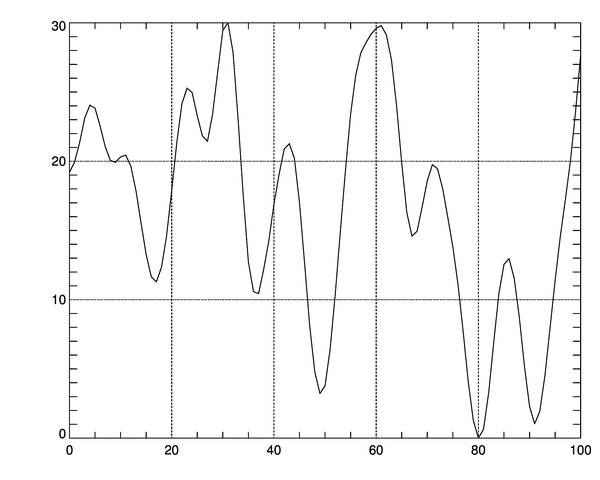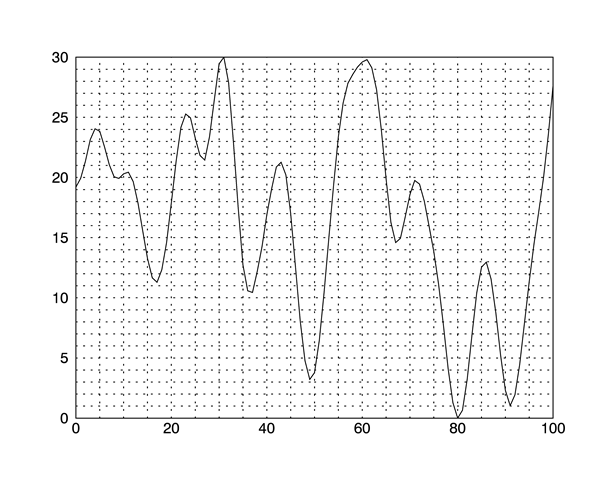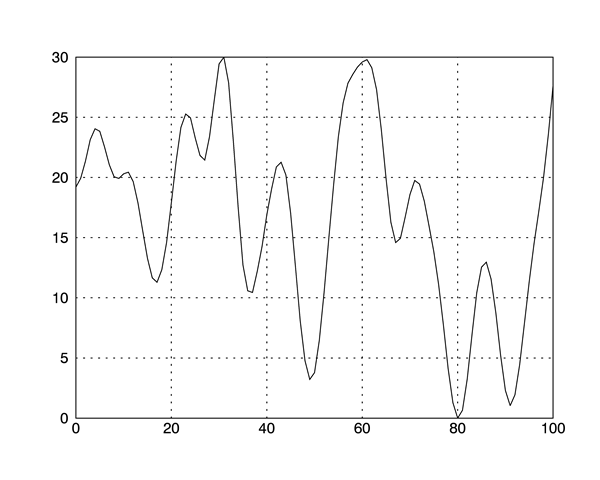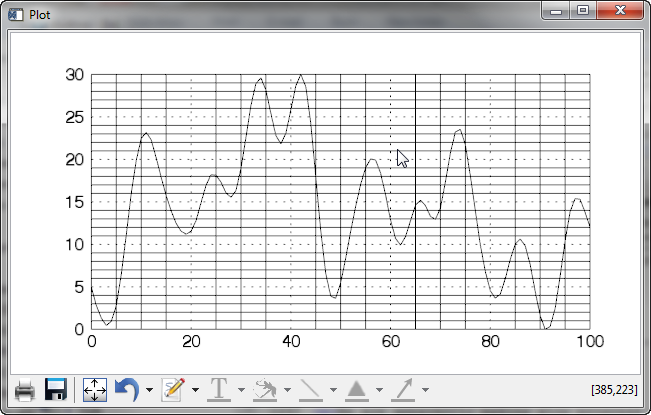Problem Creating Grid with Plot FunctionQUESTION: I am having a problem creating a grid with the IDL 8 Plot function. What I am looking for is something that looks like this direct graphics example. The documentation, such as it is, would seem to indicate that the Plot function keywords [XY]Ticklen and [XY]GridStyle work exactly like the direct graphics equivalents, but this doesn't seem to be the case. Can you help me?ANSWER: I am afraid I can't. This appears to be a bug in all IDL 8 versions up through at least IDL 8.2.1, which is the current version I am testing.

The problem seems to be that the [XY]Ticklen and [XY]GridStyle keywords are being applied to both the major and minor tick marks simultaneously.

In direct graphics, we would do something like this.

cgPlot, cgDemoData(1), XTickLen=1.0, YTickLen=1.0 , XGridStyle=1, YGridStyle=1A background grid in direct graphics.

If we try to do the same thing with the Plot function in function graphics, we get this result.

p = Plot(cgDemoData(1), XTickLen=1.0, YTickLen=1.0 , XGridStyle=1, YGridStyle=1)A background grid in function graphics.

If you can live without minor tick marks, you can, of course, suppress the minor tick marks to get a grid that is closer to what you want.

p = Plot(cgDemoData(1), XTickLen=1.0, YTickLen=1.0 , XGridStyle=1, YGridStyle=1, \$
XMinor=0, YMinor=0)A background grid in function graphics with minor ticks suppressed.Progress Report

We are apparently making slow progress with this problem. Here is the latest result in IDL 8.2.3.

p = Plot(cgDemoData(1), XTickLen=1.0, YTickLen=1.0 , XGridStyle=1, YGridStyle=1)A background grid in function graphics with IDL 8.2.3.